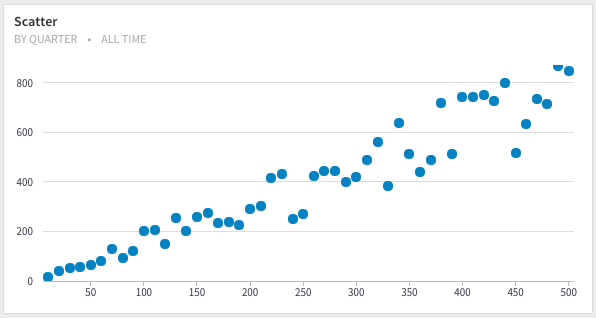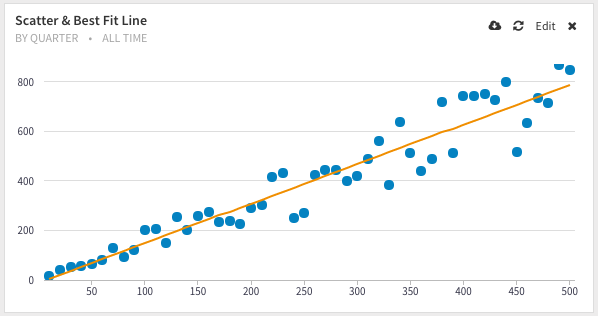# Calculating Linear Regression Coefficients in SQL

Regression is an incredibly powerful statistical tool, when used correctly, has the ability to help you predict the future. One could argue that hypothesis testing and prediction together are the pillars of data analysis.

The basic regression analysis uses fairly simple formulas to get a "best fit" line through your data. We are going to be specifically looking at simple regression analysis.

Businesses love regression analysis because it gives you powerful tools to:

• forecast next year's revenue
• predict site visitors given past historical and seasonal trends
• generate a risk score given demographics.

## The math

A regression line is simply a linethat is able to compute an output variablefor an input variable. A line can be described by two parameters, also called coefficients:

• the slope• the interceptThe coefficientsandare computed via two equations, which you can find in any textbook on statistical analysis:whereandare the averages forand.

That's it!

## The SQL

Since SQL does not have regression functions as built-ins, we are going to manually input the equations.

We are going to take an iterative procedure to constructing the coefficientsand. Let's assume we have a data in a table named ols with the columns, x and y. We first visualize the data using a scatter plot, the most common way to visually inspect the relationship between the data points:We are able to see a fairly linear relationship betweenand.

We can compute the averagesandfor all the rows in the table:

select avg(x) as x_bar,
avg(y) as y_bar
from ols;

x_bar y_bar
255 395.671

By using window functions, we can repeat the averagesandrow by row. This is to calculate the term.

select x, avg(x) over () as x_bar,
y, avg(y) over () as y_bar
from ols;


and then,

select sum((x - x_bar) * (y - y_bar)) / sum((x - x_bar) * (x - x_bar)) as slope
from (
select x, avg(x) over () as x_bar,
y, avg(y) over () as y_bar
from ols) s;

slope
1.5930700120048

To calculate the intercept, we wrap the above query in another subquery and reuse the calculation:

select slope,
y_bar_max - x_bar_max * slope as intercept
from (
select sum((x - x_bar) * (y - y_bar)) / sum((x - x_bar) * (x - x_bar)) as slope,
max(x_bar) as x_bar_max,
max(y_bar) as y_bar_max
from (
select x, avg(x) over () as x_bar,
y, avg(y) over () as y_bar
from ols) s;
)


which gives you,

slope intercept
1.5930700120048 -10.5618530612244

Hence, our best fit regression line has the equation:## Visualizing the Regression lineFor the purposes of visualizing the best fit regression line, we use the coefficients previously computed (alternatively, you can compute these coefficients on the fly):

select x, y, 1.5930700120048 * x - 10.5618530612244 as y_fit from (
select x, y from ols
) s


👋 No fuss, just SQL We are open sourcing everything from the experience working with our agency clients. They spend thousands of dollars to get this level of detailed analysis – which you can now get for free. We send one update every week. Join 400+ data analysts who are leveling up with our recipes. 👊

No spam, ever! Unsubscribe any time. See past emails here.